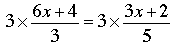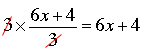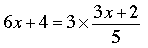SEARCH HOMEMath Central Quandaries & QueriesQuestion from Morgan, a student: Hi my math teacher has given us this question for our grade nine math class and I always get confused about multiple x algebra, could you help me? 6x+4        3x+2 ------   =  ------   3               5 I have no problems until it comes to commissioning integers, or x's ThanksHi Morgan,

First I think you should eliminate the fractions. If you multiply both sides of the equation by 3 you getYou can now cancel the two 3s on the left to getand thus.

To clear the fraction on the right, multiply both sides by 5 and cancel as above to get

5(6x + 4) = 3(3x + 2).

Using the distributive law on each side this becomes

30x + 20 = 9x + 6.

Can you complete the problem now? It may help to look at solutions to similar problems here and here.

PennyMath Central is supported by the University of Regina and The Pacific Institute for the Mathematical Sciences.